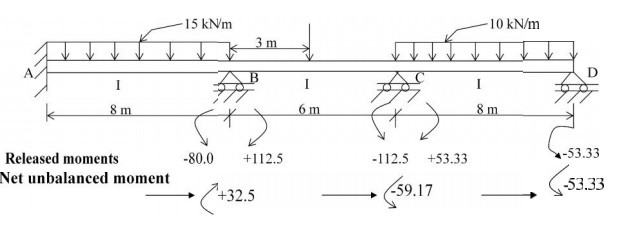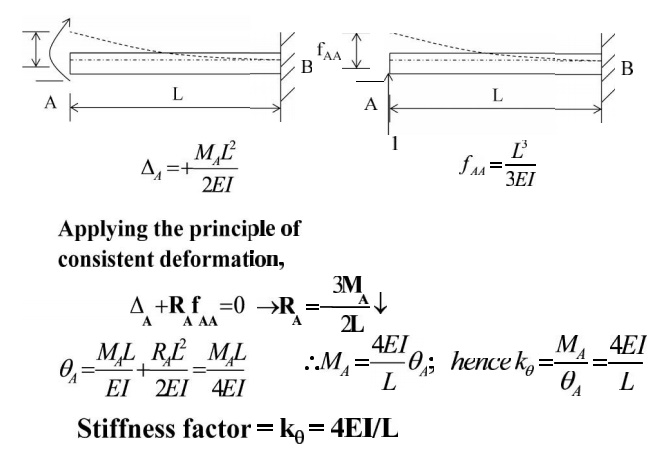Home | | Structural Analysis I | Structural Analysis - Moment Distribution Method with Solved Problems

# Structural Analysis - Moment Distribution Method with Solved Problems

The method solves for the joint mo ments in continuous beams and rigid frames by successive approxi mation

MOMENT DISTRIBUTION METHOD

INTRODUCTION AND BASIC PRINCIPLES

Introduction

(Method developed by Prof. Hardy Cross in 1932)

The method solves for the joint mo ments in continuous beams and rigid frames by successive approxi mation

Statement of Basic Principles

Consider the continuous beam ABC D, subjected to the given loads,

as shown in Figure below. Assume that only rotation of joints occur

at B, C and D, and that no support d isplacements occur at B, C and

D. Due to the applied loads in spans AB, BC and CD, rotations occur at B, C and DIn order to solve the problem in a su ccessively approximating manner,

it can be visualized to be made up of a continued two-stage problems

viz., that of locking and releasing the joints in a continuous sequence.

The joints B, C and D are locked in position before any load is applied on the b eam ABCD; then given loads are applied on the bea m. Since the joints of beam ABCD are locke d in position, beams AB, BC and CD acts as ind ividual and separate fixed beams, subjected to the applied loads; these loads develop fixed ennd moments.In beam AB

Fixed end moment at A = -wl2/12 = - (15)(8)(8)/12 = - 80 kN.m

Fixed end moment at B = +wl2/12 = +(15)(8)(8)/12 = + 80 kN.m

In beam BC

Fixed end moment at B = - (Pab2)/l2 = - (150)(3)(3)2/62

= -112.5 kN.m

Fixed end moment at C = + (Pab2)/l2 = + (150)(3)(3)2/62

= + 112.5

In beam AB

Fixed end moment at C = -wl2/12 = - (10)(8)(8)/12 = - 53.33 kN.m

Fixed end moment at D = +wl2/12 = +(10)(8)(8)/12 = + 53.33kN.m

Since the joints B, C and D were fix ed artificially (to compute the the fixed-end moments), now the joints B, C and D are released and allowed to rotate. Due to the  joint release, the joints rotate maintaining the continuous nature of the beam. Due to the joint release, the fixed en d moments on either side of joints B, C and D act in the opposite direction now, and cause a net un balanced moment to occur at the joint.These unbalanced moments act at th e joints and modify the joint moments at B, C a nd D, according to their relative stiffnesses at the respective joints. The joint moments are distributed t o either side of the joint B, C or D, according to their reelative stiffnesses. These distributed moments al so modify the moments at the opposite side of the beam span, viz., at joint A in span AB, at joints B and C in span BC and at joints C and D in span CD. This modification is dependent on the carry-over factor (which is equal to 0.5 in this case);

The carry-over moment becomes the unbalanced moment at the joints to whic h they are carried over. Steps 3 and 4 are repeated t ill the carry-over or distributed moment beco mes small.

Sum up all the moments at each of the joint to obtain the joint moments.

SOME BASIC DEFINITIONS

In order to understand the five steps mentioned in section 7.3, some words need to be defined and relevant derivations made.

1Stiffness and Carry-over Factors

Stiffness = Resistance offered by m ember to a unit displacement or rotation at a point, for given support constraint conditionsA clockwise moment MA is applied at A to produce a +ve bending in beam AB. Fin d qA and MB.

Using method of consistent defor mationsConsidering moment MB,

MB + MA + RAL = 0

\MB = MA/2= (1/2)MA

Carry - over Factor = 1/2

2 Distribution Factor

Distribution factor is the ratio according to which an externally applied unbalanced moment M at a joint is apportioned to the various m embers mating at the jointM = MBA + MBC + MBDModified Stiffness Factor

The stiffness factor changes when t he far end of the beam is simply-supported.As per earlier equations for deforma tion, given in Mechanics of Solids text-books.Solve the previously given proble m by the moment distribution method

Fixed end momentsStiffness Factors (Unmodified StifffnessDistribution FactorsComputation of Shear ForcesStudy Material, Lecturing Notes, Assignment, Reference, Wiki description explanation, brief detail
Civil : Structural Analysis : Moment Distribution Method : Structural Analysis - Moment Distribution Method with Solved Problems |